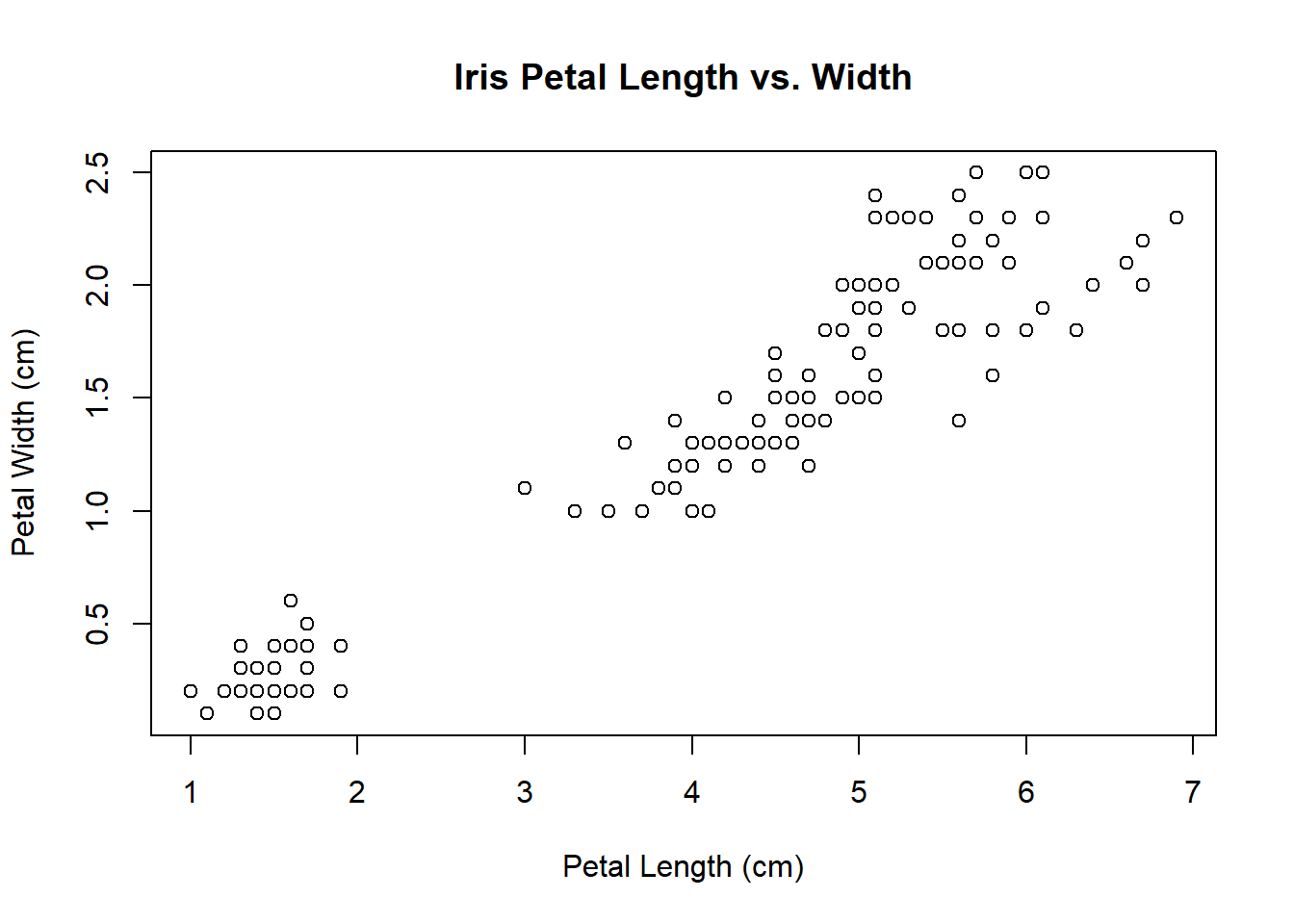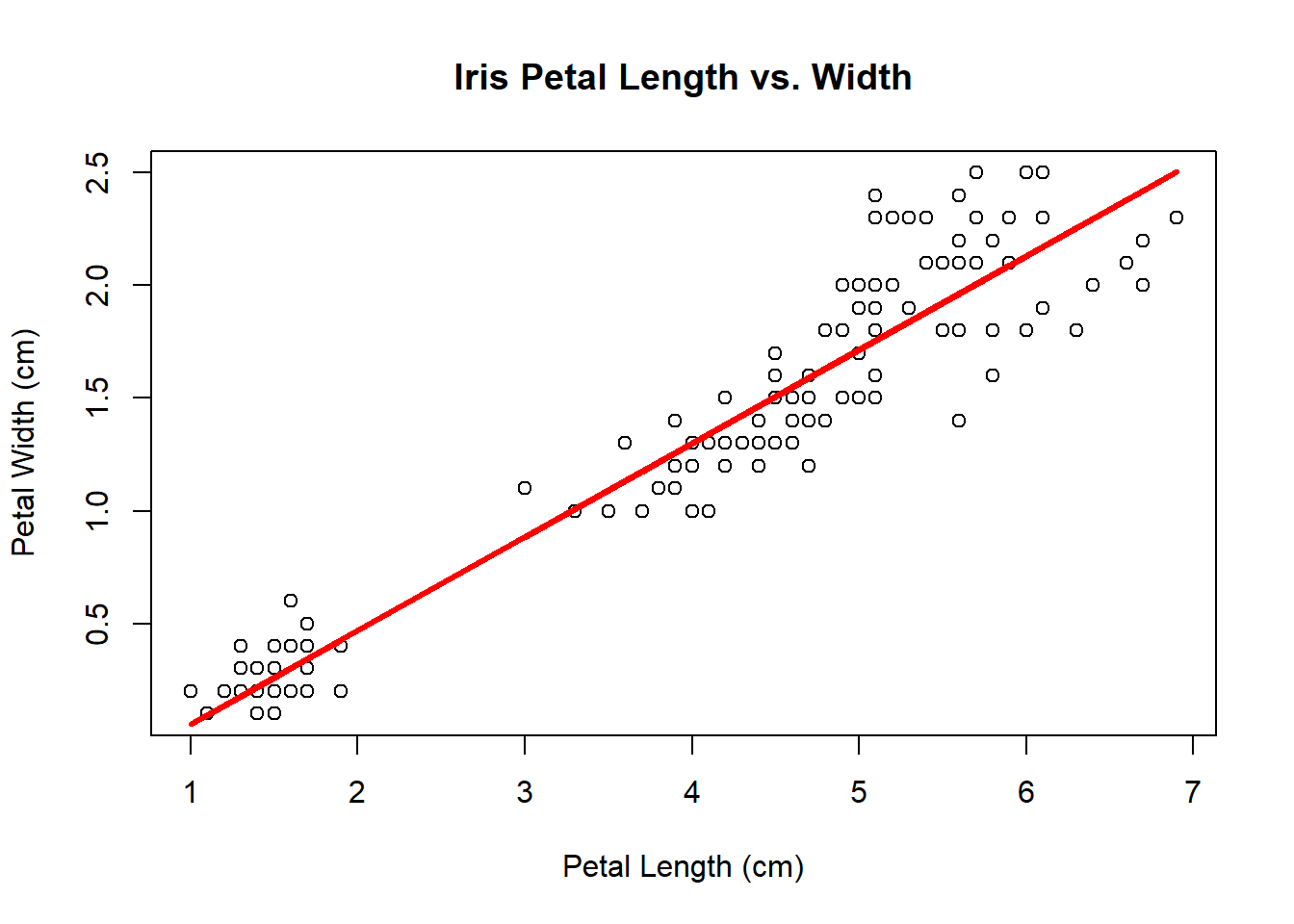# Lab 6A: Handling Big Data (Easy)

1. Set working directory
setwd("C:/Workshop/Data")
library(ff)
1. Read a CSV file as ff data frame
irisff <- read.table.ffdf(
file = "Iris.csv",
FUN = "read.csv")
1. Inspect the class
class(irisff)
##  "ffdf"
1. Inspect the column names
names(irisff)
##  "Sepal.Length" "Sepal.Width"  "Petal.Length" "Petal.Width"
##  "Species"
1. Inspect the first few rows
irisff[1:5,]
##   Sepal.Length Sepal.Width Petal.Length Petal.Width Species
## 1          5.1         3.5          1.4         0.2  setosa
## 2          4.9         3.0          1.4         0.2  setosa
## 3          4.7         3.2          1.3         0.2  setosa
## 4          4.6         3.1          1.5         0.2  setosa
## 5          5.0         3.6          1.4         0.2  setosa
library(biglm)
1. Create a linear regression model
model <- biglm(
formula = Petal.Width ~ Petal.Length,
data = irisff)
1. Summarize the model
summary(model)
## Large data regression model: biglm(formula = Petal.Width ~ Petal.Length, data = irisff)
## Sample size =  150
##                 Coef    (95%     CI)     SE p
## (Intercept)  -0.3631 -0.4426 -0.2836 0.0398 0
## Petal.Length  0.4158  0.3966  0.4349 0.0096 0
1. Create a scatterplot
plot(
x = irisff$Petal.Length[], y = irisff$Petal.Width[],
main = "Iris Petal Length vs. Width",
xlab = "Petal Length (cm)",
ylab = "Petal Width (cm)")1. Get y-intercept from model
b <- summary(model)$mat[1,1] 1. Get slope from model m <- summary(model)$mat[2,1]
1. Draw a regression line on plot
plot(
x = irisff$Petal.Length[], y = irisff$Petal.Width[],
main = "Iris Petal Length vs. Width",
xlab = "Petal Length (cm)",
ylab = "Petal Width (cm)")

lines(
x = irisff$Petal.Length[], y = m * irisff$Petal.Length[] + b,
col = "red",
lwd = 3)1. Predict new values with the model NOTE: BigLM requires zeros for Petal.Width
predict(
object = model,
newdata = data.frame(
Petal.Length = c(2, 5, 7),
Petal.Width = c(0, 0, 0)))
##        [,1]
## 1 0.4684353
## 2 1.7157016
## 3 2.5472124